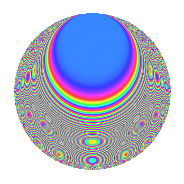# Properties

 Label 1344.2.iLevel 1344 Weight 2 Character orbit i Rep. character $$\chi_{1344}(545,\cdot)$$ Character field $$\Q$$ Dimension 64 Newforms 7 Sturm bound 512 Trace bound 21

# Related objects

## Defining parameters

 Level: $$N$$ = $$1344 = 2^{6} \cdot 3 \cdot 7$$ Weight: $$k$$ = $$2$$ Character orbit: $$[\chi]$$ = 1344.i (of order $$2$$ and degree $$1$$) Character conductor: $$\operatorname{cond}(\chi)$$ = $$168$$ Character field: $$\Q$$ Newforms: $$7$$ Sturm bound: $$512$$ Trace bound: $$21$$ Distinguishing $$T_p$$: $$5$$, $$13$$, $$19$$, $$29$$

## Dimensions

The following table gives the dimensions of various subspaces of $$M_{2}(1344, [\chi])$$.

Total New Old
Modular forms 280 64 216
Cusp forms 232 64 168
Eisenstein series 48 0 48

## Trace form

 $$64q$$ $$\mathstrut +\mathstrut O(q^{10})$$ $$64q$$ $$\mathstrut -\mathstrut 64q^{25}$$ $$\mathstrut -\mathstrut 16q^{49}$$ $$\mathstrut -\mathstrut 96q^{81}$$ $$\mathstrut +\mathstrut O(q^{100})$$

## Decomposition of $$S_{2}^{\mathrm{new}}(1344, [\chi])$$ into irreducible Hecke orbits

Label Dim. $$A$$ Field CM Traces $q$-expansion
$$a_2$$ $$a_3$$ $$a_5$$ $$a_7$$
1344.2.i.a $$4$$ $$10.732$$ $$\Q(\zeta_{12})$$ $$\Q(\sqrt{-3})$$ $$0$$ $$0$$ $$0$$ $$0$$ $$q+\zeta_{12}q^{3}+(\zeta_{12}-\zeta_{12}^{3})q^{7}+3q^{9}+\cdots$$
1344.2.i.b $$4$$ $$10.732$$ $$\Q(\zeta_{12})$$ $$\Q(\sqrt{-3})$$ $$0$$ $$0$$ $$0$$ $$0$$ $$q+\zeta_{12}q^{3}+(-\zeta_{12}+\zeta_{12}^{3})q^{7}+3q^{9}+\cdots$$
1344.2.i.c $$8$$ $$10.732$$ 8.0.49787136.1 $$\Q(\sqrt{-42})$$ $$0$$ $$0$$ $$0$$ $$0$$ $$q-\beta _{1}q^{3}+\beta _{3}q^{7}-3q^{9}+\beta _{7}q^{13}+\cdots$$
1344.2.i.d $$8$$ $$10.732$$ 8.0.40960000.1 None $$0$$ $$0$$ $$0$$ $$0$$ $$q-\beta _{5}q^{3}+(-2\beta _{2}-\beta _{7})q^{5}+(-\beta _{3}+\cdots)q^{7}+\cdots$$
1344.2.i.e $$8$$ $$10.732$$ 8.0.40960000.1 None $$0$$ $$0$$ $$0$$ $$0$$ $$q-\beta _{5}q^{3}+(-2\beta _{2}-\beta _{7})q^{5}+(-\beta _{3}+\cdots)q^{7}+\cdots$$
1344.2.i.f $$16$$ $$10.732$$ 16.0.$$\cdots$$.1 None $$0$$ $$-16$$ $$0$$ $$0$$ $$q+(-1+\beta _{6})q^{3}+\beta _{5}q^{5}-\beta _{2}q^{7}+(-1+\cdots)q^{9}+\cdots$$
1344.2.i.g $$16$$ $$10.732$$ 16.0.$$\cdots$$.1 None $$0$$ $$16$$ $$0$$ $$0$$ $$q+(1+\beta _{6})q^{3}+\beta _{5}q^{5}+\beta _{3}q^{7}+(-1+\cdots)q^{9}+\cdots$$

## Decomposition of $$S_{2}^{\mathrm{old}}(1344, [\chi])$$ into lower level spaces

$$S_{2}^{\mathrm{old}}(1344, [\chi]) \cong$$ $$S_{2}^{\mathrm{new}}(168, [\chi])$$$$^{\oplus 4}$$$$\oplus$$$$S_{2}^{\mathrm{new}}(672, [\chi])$$$$^{\oplus 2}$$